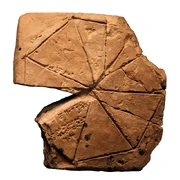## FANDOM

902 Pages

The History of Mathematics- A BBC Documentary

Patterns in the UniverseGeometry problem on a clay tablet belonging to a school for scribs; Susa, first half of the 2nd millennium BCE Origin in ancient Egypt. Egyptians develop fractions for

practical needs, e.g. to divide up 9 loafs of bread equally between 10 people. No general theories in Egyptian mathematics, only concrete numbers.

From Egypt to Babylonia. Base of 60 – from Babylonia. E.g. in time. Invented 0.

Greek math. Invent a deductive system: axioms and proof. Pythagoras in Samos. Schools were “a way of life”: communal living, involved in city politics. Appeal to beautiful arguments. Relationship between a good solution and beauty.

Plato considers math as bedrock of knowledge. Geometry is the key to understanding the Universe. Understanding of geometry required for philosophy.

Euclid – a system of axioms and proofs. Euclidian geometry. Alexandria. Archimedes. Designed weapons. Calculating the area of an unknown figure using a known figure: calculating the area of a circle using triangle approximation.

BBC The Story of Maths - The Genius of the East FULL (2of4)

China: started building the Great Wall in 220 B.C., stimulates mathematics about volumes (of materials), angles, etc. Use decimal place value system, i.e. a units column, a tens columns, a hundreds, etc.

India – invent “0” in 9th century A.D.

Trigonometry – translates shapes (geometry) into numbers and back. Math is the study of number and form. Number and form are the opposites tied together.

Trigonometry used for understanding the distances in space, also in engineering and sailing.

Islamic world – in Baghdad, “the house of Wisdom”. Cubic equations. Omar Khayam – a poet and a mathematician, tries to find a general solution to cubic (or quadratic?) equations.

Feudal Europe, Italy – Fibonacci (Leonardo of Pizza). Introduced Hindu-Arabic numerals to Europe, against the opposition of conservatives who saw this as too easy (as opposed to Roman numerals), and thus giving power to the masses. Fibonacci numbers – used for calculating growth.

Tartaglia – in Bologna. Slashed across the face by a French army. Finds a formula to solve all kinds of cubic equations.

BBC The Story of Maths - The Frontiers of Space (3of4)

combination of opposite qualities in a person, e.g. a mathematician and an artist.

Descartes links geometry to algebra using the Cartesian coordinates, and writing equations

Fermat – invents the modern number theory

(Development of mathematics, and science, knowledge in general coincides with development of this or that type of culture.)

Newton – calculus

Leibniz (as Descartes) liked to lie in the bed in the morning to think, to jot down his ideas. Leibniz invented a practical calculating machine.

Bernoulli – from Basel, Switzerland. Merchants.

Leonard Euler – a protégé of Bernoulli

(Humboldt – a great educationist in Germany, XVIII century)

France, XVIII century – Fourier analysis.

Germany, XVIII century – Gauss. First idea that the space is curved. Didn’t dare to publish.

Janos Bolyai – “hyperbolic geometry”, angles of a triangle add up to less than 180 degrees

Lobachevsky discovered the same geometry 2 years prior to Bolyai.

B. Reimann – anticipates development of relativity theory. Mathematics in general anticipates developments in science and technology. We have the math, but don’t know its meaning in the real world. Math is an abstraction.

BBC The Story of Maths - To Infinity and Beyond (4of4)

Galois (1832) – mathematics is not a study of numbers or shapes, but of structures.

“A mathematician is a pattern searcher”

Hilbert proves that all equations can be derived from a finite set of equations. What the set was he couldn’t tell. Liked to flirt with young women, ride bike, drink with students. Hilbert sets a number of math problems.

Cantor – explores different kinds of infinities. Compares their sizes. Suffers from manic depression (a psychiatric diagnosis for a mood disorder in which people experience disruptive mood swings that encompass a frenzied state known as mania (or hypomania) and, usually,symptoms of depression… у больного наблюдаются быстрая смена симптомов мании (гипомании) и депрессии, либо симптомы депрессии и мании одновременно (например, тоска со взвинченностью, беспокойством либо эйфория с заторможенностью — так называемая непродуктивная мания — или другие)

Henri Poincare – French mathematician, supported Cantor. 2 hours of work in the morning, and 2 hours of work in the early evening. In between – subconscious thinking.

Chaos theory

Topology – started as the problem of “7 bridges of Konigsberg”

Kurt Godel – an odd guy, part of “Vienna circle” group of philosophers. Set out to solve Hilbert’s second problem-challenge. Finds out that in any logical system (in mathematics only?) there will be statements that are true but which one cannot prove within the system. (25 min. in the video). Attacked by Nazi thugs. Flees to the research center of the Institute for Advanced Study in Princeton.

Paul Cohen – an American mathematician in 1950’s. Resolves the problem of Cantor of whether there is an infinity between the whole numbers and fractions.

(Lesson: if there is a problem that appears unsolvable, need to take it to a higher level, i.e. look wider than the immediate problem.)

Julia Robertson – first woman to be elected of the American Mathematics Society. 10th problem of Hilbert – is there a universal method for telling whether any equation has a whole number solution, or not?

Yuri Matusevich – at 22 solves Hilbert’s 10th problem. Steklov Institute in St. Petersrburg.

Poincare conjecture – solved in 2002 by G. Perelman, St. Petersburg. Not accessible for interview. Solves the problem of Poincare by connecting it to another problem, i.e. by combining problems. This seems like a common trick.

History of Mathematics : Mathematics Time Line

Brief (2,5 min.) with subtitles, a good intro

XX century: Touring, Newmann

History of mathematics